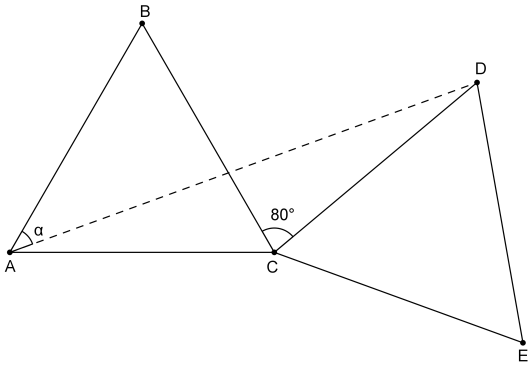# Rotating Triangles

Geometry Level 1

Let $\triangle ABC$ and $\triangle CDE$ be equilateral triangles of the same size, and $\angle BCD=80^\circ$ between them. Find the measure of $\angle BAD$ in degrees.×